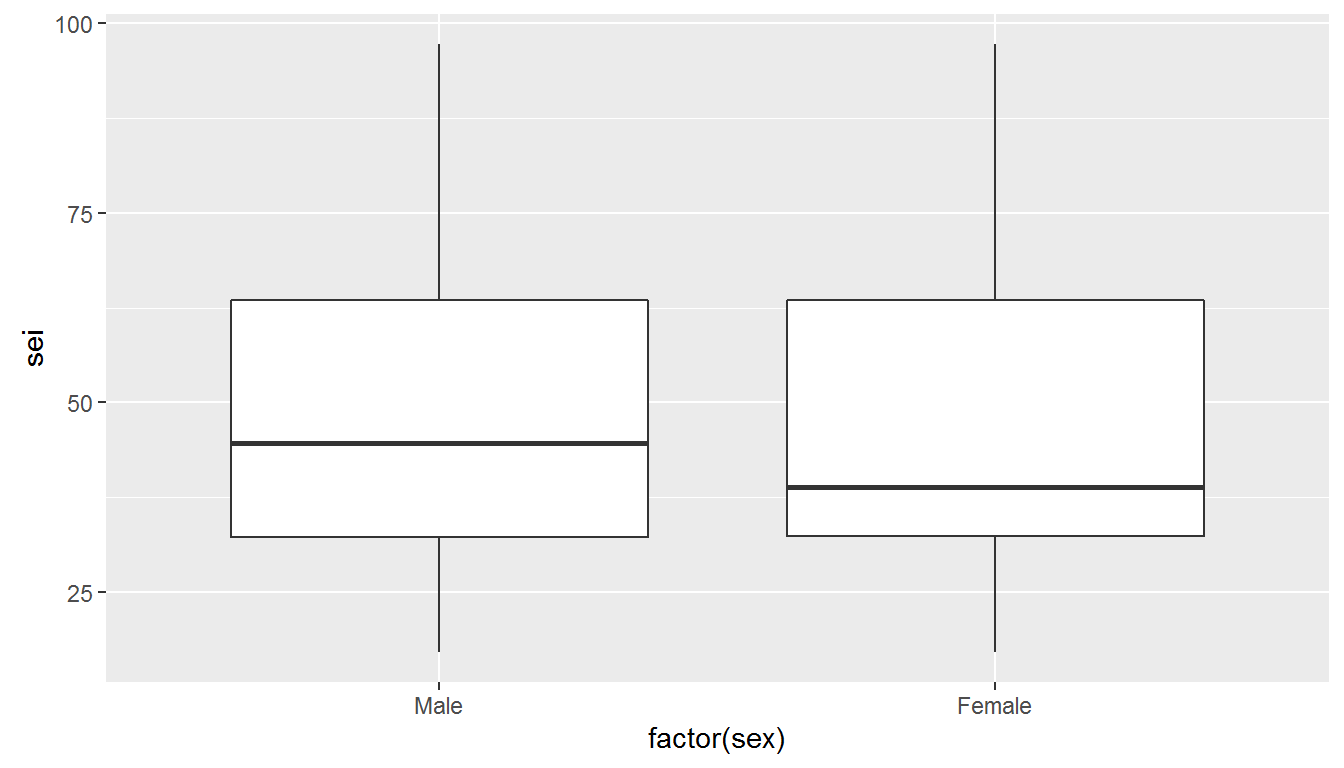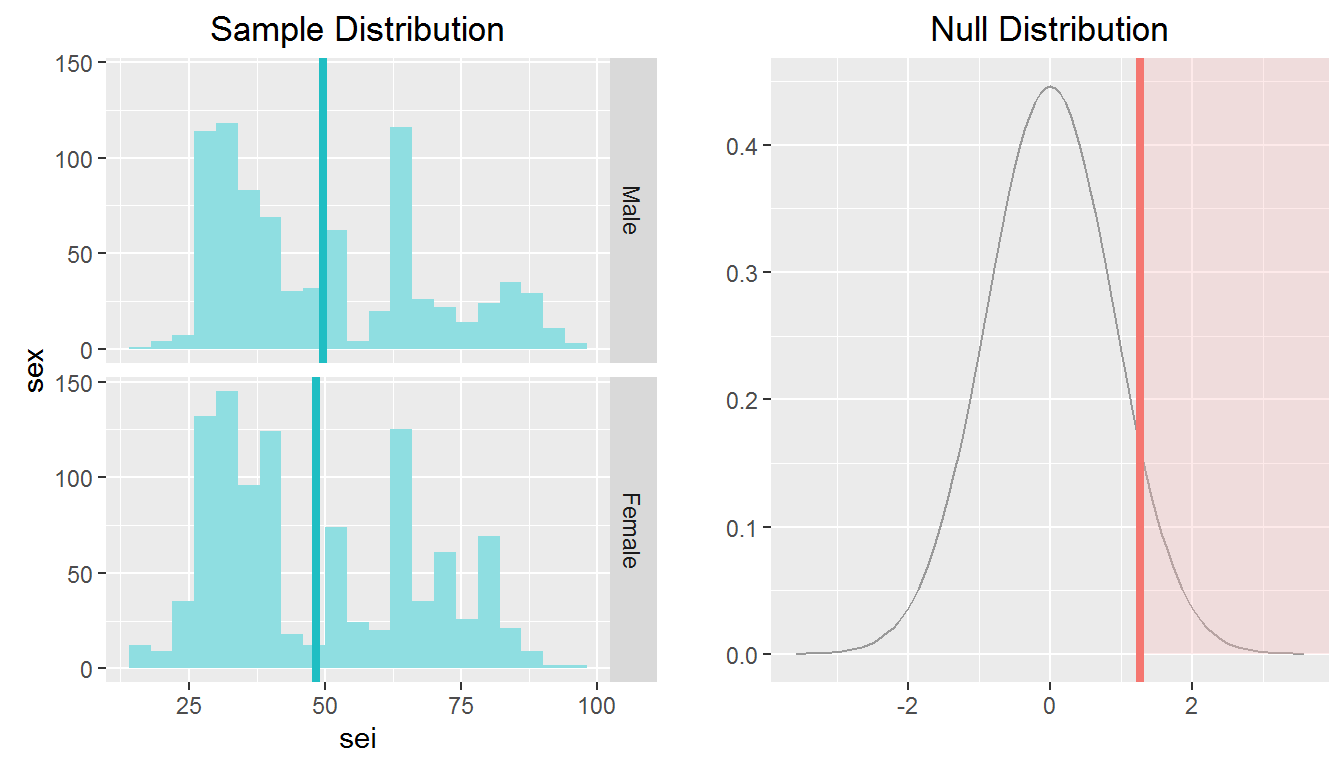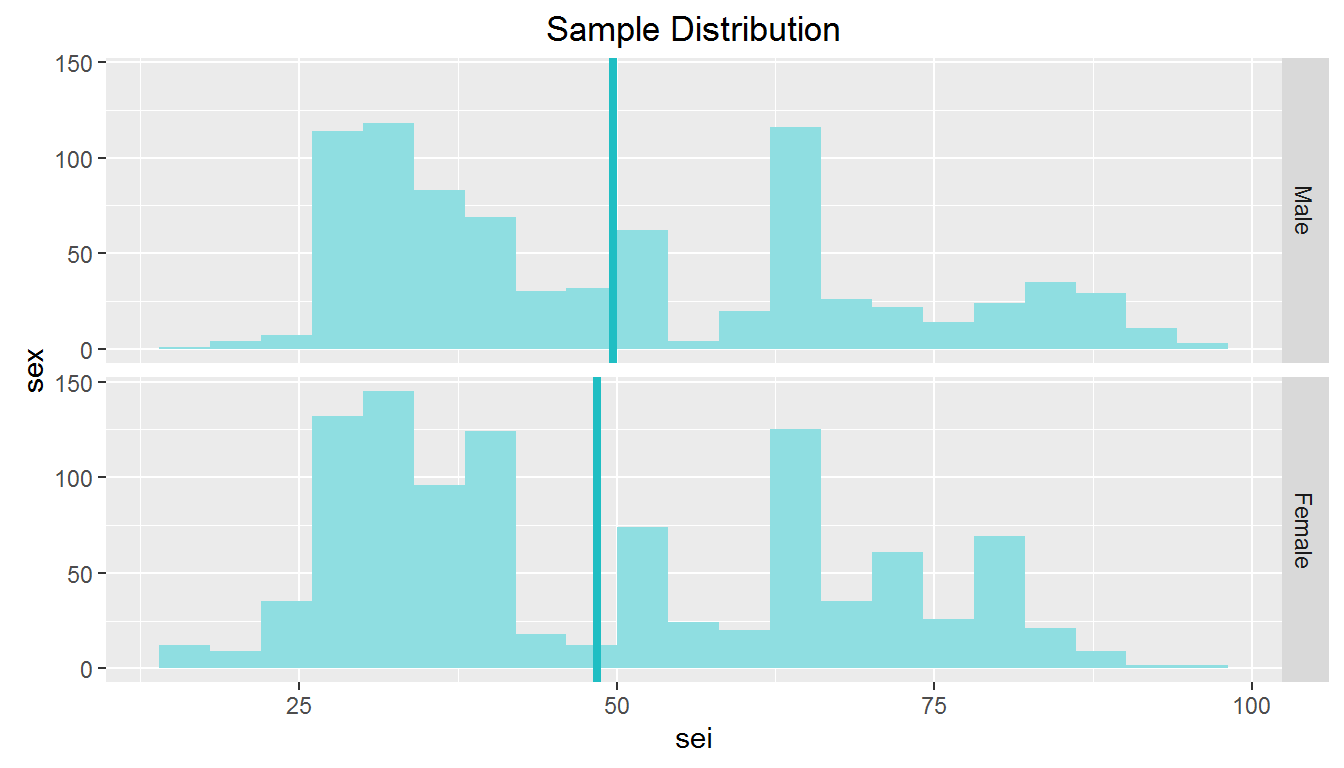Setup

library(ggplot2)
## Warning: package 'ggplot2' was built under R version 3.3.1
library(dplyr)
## Warning: package 'dplyr' was built under R version 3.3.1
library(statsr)

Part 1: Data

The General Social Survey (GSS) is a sociological survey used to collect information and keep a historical record of the concerns, experiences, attitudes, and practices of residents of the United States.

Data Collection: The vast majority of GSS data is obtained in face-to-face interviews. Computer-assisted personal interviewing (CAPI) began in the 2002 GSS. Under some conditions when it has proved difficult to arrange an in-person interview with a sampled respondent, GSS interviews may be conducted by telephone.

Sampling: The GSS sample is drawn using an area probability design that randomly selects respondents in households across the United States. Respondents that become part of the GSS sample are from a mix of urban, suburban, and rural geographic areas.

Generalizability: The inferences made from this data set are generalizable because respondents are randomly selected. The results are generalizabile to the GSS Target population, which is Adults (18+) living in households in the United States. Residents of institutions and group quarters are out-of-scope.

Causality: This is an observational study with no random assignment. We cannot infer causation.

Part 2: Research question

Is the average 2010 Socioeconomic (SEI) score for men higher than it is for women? I will use the Sex and SEI variables to assess how the mean scores for men and women compare for 2010, the most recent year with SEI scores.

Socioeconomic index (SEI) scores summarize the differences in prestige between occupations, as assessed by the education required and the earnings provided. It is commonly conceptualized as the social standing or class of an individual or group. It will be interesting to see if the mean SEI for men is higher than women. If true it providers some evidence that continued investment in programs driving and promoting the equality of women is needed.

Part 3: Exploratory data analysis

Selected_GSSData <- gss %>% filter(!(is.na(sei)))

I noticed not all years have SEI index scores. I first select the records that have an associated SEI score.

Selected_GSSData %>%
group_by(year) %>%
summarise(mean_sei = mean(sei))
## Source: local data frame [14 x 2]
##
##     year mean_sei
##    <int>    <dbl>
## 1   1988 45.60534
## 2   1989 46.76194
## 3   1990 46.70526
## 4   1991 46.27347
## 5   1993 47.19314
## 6   1994 47.33987
## 7   1996 47.85451
## 8   1998 49.13881
## 9   2000 49.11847
## 10  2002 49.21335
## 11  2004 50.80449
## 12  2006 49.40959
## 13  2008 48.76002
## 14  2010 48.99232

I next look at mean scores across the years. Generally the means score seems to be increasing from 45.60534 in 1988 to 48.99232 in 2010.

gss2010 <- Selected_GSSData %>%
filter(year == "2010")

I decided to focus just on the most recent yea, 2010, to have the most current scores available and to not have average influenced by previous years. There are also enough observations (n=1875).

summary(gss2010\$sei)
##    Min. 1st Qu.  Median    Mean 3rd Qu.    Max.
##   17.10   32.40   41.20   48.99   63.50   97.20

This summary shows the Median 2010 SEI score is 41.2 with a max of 97.2 and a min of 17.1. We know that50% of the scores would fall in a range of 32.4 (Q1) to 63.5 (Q3)

ggplot(gss2010, aes(x = factor(sex), y = sei)) +
geom_boxplot()Side by side box plots are a good way to initially look for any relationships between Sex and SEI score. The graphic suggests there is a difference between sEI scores for Men and Women in 2010. The Median SEI for Men is higher than than Median SEI for Women, but we don’t know if this is signficant.

gss2010 %>%
group_by(sex) %>%
summarise(mean_sei = mean(sei))
## Source: local data frame [2 x 2]
##
##      sex mean_sei
##   <fctr>    <dbl>
## 1   Male 49.70413
## 2 Female 48.43425

This summary shows mean SEI scores by sex. The mean SEI score for men is 49.70413 and the mean for Women is 48.43425. The means are different, but I don’t know if this is a statistical difference.

Part 4: Inference

Based on my exploratory data analysis, I’ve decided to form my hypothesis to assess if mean Male SEI scores are higher than female. The data suggested male SEI scores are higher based on the box plots and summary statistics.

HO: MU_MaleSEI = MU_FemaleSEI HA: MU_MaleSEI > MU_FemaleSEI

My Null Hypothses states there is NO difference in the SEI means between Males and Females - they are essentially equal.

My Alternative Hypotheses (the one I am testing) says Male SEI means are higher than Female SEI means.

My parameter of interest is the difference between Male and Female SEI means or 1.26988.

For my Hypothesis test, I have One Numerical (SEI means) and One Categorical with two levels (Sex - Male-Female). I am chosing to do a One Sided T-Test for the difference of means for Two Indepedent Samples.

This Hypothesis test will determine if there is a statisticially signifcant difference in the mean SEI scores for Males and Females. My hypothesis is the Male mean SEI is greater than the Female mean SEI, which is why I am doing a one tailed test. The test will assign a P value to the Tscore of the difference. This P score will show the probability of getting a difference as large as 1.26988 if in fact the Null Hypothesis of no difference is True.

I chose an Alpha level of 0.05. If the Test determines the probability of getting a difference of 1.26988 assuming the Null is true is less than .05, I will conclude there is in fact a significant difference. Because this is a one sided test assessing if the Male mean score is Higher, it will only be looking for probability of getting values equal to or higher than the observed difference of 1.26988.

Conditions

I need to make sure my data is appropriate (YES/NO) for this test by checking conditions:

Independence WITHIN BOTH Male and Female samples. YES-Satisfied.

1.) Random Selection: SATISFIED BOTH SAMPLES. GSS stated respondents are recruited randomly and thus we meet this condition for both Male and Female samples.

2.) Sample Size <10% of total US Males and Females assuming sampling without replacement: SATISFIED BOTH SAMPLES Males (n=824) and Females (n=1051) are both less than all Males and Females in the US.

3.) Independence BETWEEN BOTH Male and Female samples. YES. The two groups are indepedent. No mention they are married and I am going to assume they are NOT. This is reinforced by there is only 1 respondent per household.

Sample Size Skew YES-Satisfied: The sample size for both groups is in the hundreds and the box plot showed relatively normal distributions. Neither group had a lot of outliers. I am not concerned by the distribution being overly skewed or not having enough sample observations.

inference(y = sei, x = sex, data = gss2010, statistic = "mean", type = "ht", null = 0,
alternative = "greater", method = "theoretical")
## Response variable: numerical
## Explanatory variable: categorical (2 levels)
## n_Male = 824, y_bar_Male = 49.7041, s_Male = 19.4623
## n_Female = 1051, y_bar_Female = 48.4343, s_Female = 18.9112
## H0: mu_Male =  mu_Female
## HA: mu_Male > mu_Female
## t = 1.4198, df = 823
## p_value = 0.078The T test associated with the difference is 1.4198, which corresponds to a P value of 0.078. The P value is NOT small and NOT below my target Alpha of 0.05. I

I CANNOT reject my null Hypothesis stating there is NO difference in Male and Female mean SEI scores.

inference(y = sei, x = sex, data = gss2010, statistic = "mean", type = "ci",  method = "theoretical")
## Response variable: numerical, Explanatory variable: categorical (2 levels)
## n_Male = 824, y_bar_Male = 49.7041, s_Male = 19.4623
## n_Female = 1051, y_bar_Female = 48.4343, s_Female = 18.9112
## 95% CI (Male - Female): (-0.4857 , 3.0255)I can also do a Confidence Interval (C.I.), because my parameter of interest is numerical (the difference in Male and Female mean SEI scores) I can take my point estimate and create a 95% C.I.

The results of the 95% Confidence Interval also support the Hypothesis test. Based on this test I am 95% confident the difference in Male and Female mean SEI is (-0.4857,3.0255). The Null Hypothesis value of “0” or No difference is included in this interval.

Final Conclusions: I was unable to reject my Null hypothesis of there being NO difference between Male and Female mean SEI scores, which means the two averages are the same statistically and the difference is due to random error. I do not have support mean Male SEI scores are higher than Female.

This provides some evidence there is not a significant disparity between Male and Female Socio Economic status in the US for 2010. I am not able to say anthing about what causes these results. However, this might be interesting further analysis to pursue.